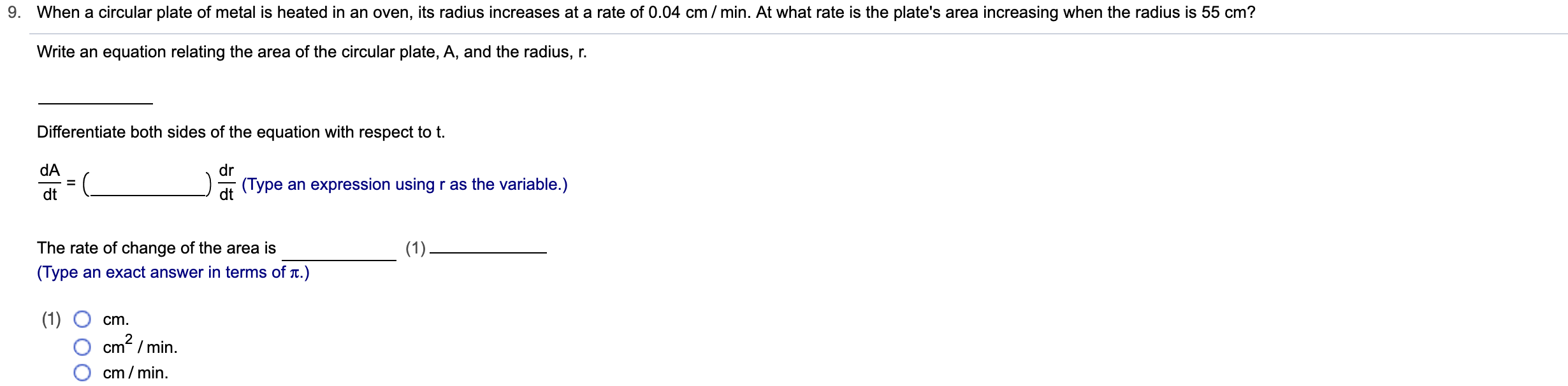# When a circular plate of metal is heated in an oven, its radius increases at a rate of 0.04 cm / min. At what rate is the plate's area increasing when the radius is 55 cm?Write an equation relating the area of the circular plate, A, and the radius, rDifferentiate both sides of the equation with respect to t.dAdr(Type an expression using r as the variable.)dtThe rate of change of the area is(1)(Type an exact answer in terms of t.)(1)cm2/mincmcm/min

Question
31 views

Can you help me step by step?help_outlineImage TranscriptioncloseWhen a circular plate of metal is heated in an oven, its radius increases at a rate of 0.04 cm / min. At what rate is the plate's area increasing when the radius is 55 cm? Write an equation relating the area of the circular plate, A, and the radius, r Differentiate both sides of the equation with respect to t. dA dr (Type an expression using r as the variable.) dt The rate of change of the area is (1) (Type an exact answer in terms of t.) (1) cm2/min cm cm/min fullscreen
check_circle

Step 1

Given a circular plate is heated in an oven such that its radius (r) increases at a rate of 0.04 cm/min that is

Step 2

The equation relating the area A, and the radius r is

Step 3

Differentiating both side of ...

### Want to see the full answer?

See Solution

#### Want to see this answer and more?

Solutions are written by subject experts who are available 24/7. Questions are typically answered within 1 hour.*

See Solution
*Response times may vary by subject and question.
Tagged in

### Other